Mesons are combinations of two quarks.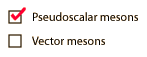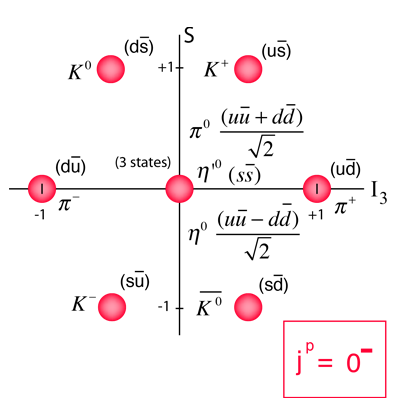The pseudoscalar mesons have quark and antiquark spins antialigned and zero orbital angular momentum, resulting in j=0. They have negative parity. The diagrams are plotted with strangeness on the vertical axis and isospin on the horizontal axis.

 Table of mesons
Index

Particle concepts

 HyperPhysics***** Quantum Physics R Nave
Go BackMesons are combinations of two quarks.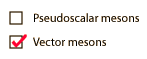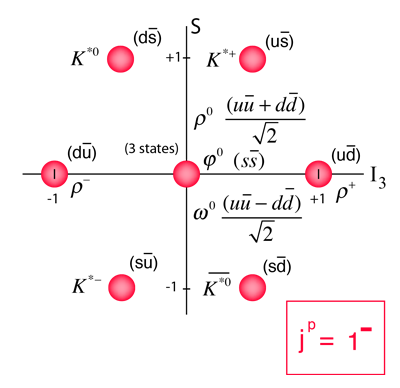The vector mesons have quark and antiquark spins aligned and zero orbital angular momentum, resulting in j=1. They have negative parity. The diagrams are plotted with strangeness on the vertical axis and isospin on the horizontal axis.

The rho vector meson has a mass dramatically larger than the pseudoscalar pi meson which has the same quark content, illustrating the extraordinary variation of effective quark masses.

 Table of mesons
Index

Particle concepts

 HyperPhysics***** Quantum Physics R Nave
Go Back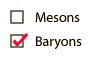Baryons are combinations of three quarks.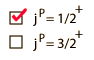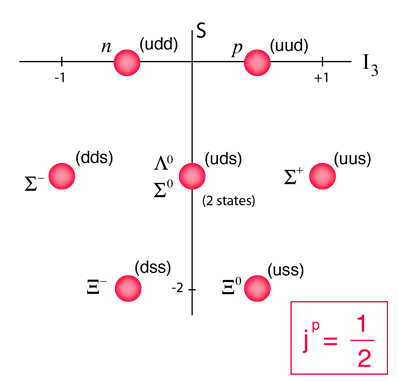The diagrams are plotted with strangeness on the vertical axis and isospin projection on the horizontal axis.

### Table of Baryons

Index

Particle concepts

 HyperPhysics***** Quantum Physics R Nave
Go BackBaryons are combinations of three quarks.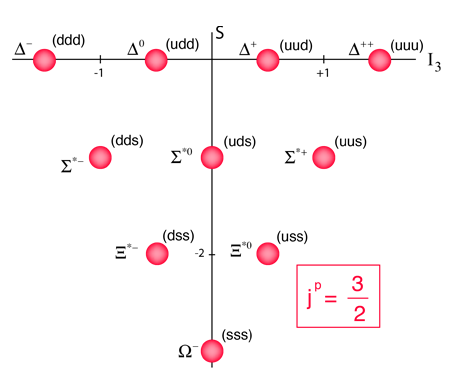The diagrams are plotted with strangeness on the vertical axis and isospin projection on the horizontal axis.

### Table of Baryons

Index

Particle concepts

 HyperPhysics***** Quantum Physics R Nave
Go Back